Contact Home

## Procedurally Generated Trees with Space Colonization Algorithm in XNA C#

I discovered a very interesting algorithm called “Space Colonization Algorithm”, which is described in a paper found here:

http://algorithmicbotany.org/papers/colonization.egwnp2007.html

With this algorithm, it is possible to create some pretty lifelike trees. With L-Systems, you can get some interesting looking trees, which typically start from the root, and generates branches based on a set of rules. The Space Colonization algorithm takes a different approach to the problem. Instead you first create the leaves, which serve as attraction points for the branches. Over each iteration, the branches grow closer and closer towards the leaves. In this manner, you end up having tree branches that appear to have grown towards some light source.

I decided to write the algorithm in C#. The following is an example of a tree that was generated using this algorithm: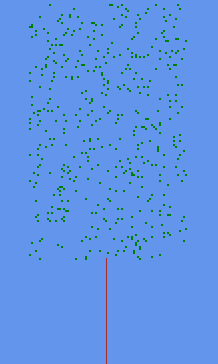The algorithm works as follows:

1. Define an area for the crown of the tree. In our examples, we are using Rectangles, but any shape can be used. In fact, very interesting trees can be created by using various shapes.
2. Populate the defined area with attraction points (I call these leaves).
3. Create the trunk of the tree, by adding Branches below the defined area. Keep growing branches upwards until the MaxDistance between a Leaf and a Branch is reached. This MaxDistance is a parameter that defines how far a Leaf can be to attract a Branch. A Branch is not affected by Leaves that are further away than MaxDistance.
4. At this point, your tree would look something like this, and is now ready to grow: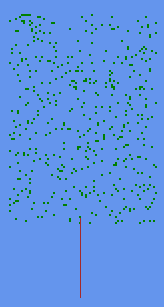5. Process the Leaves, by comparing it to all the Branches. Calculate the direction and distance from the Leaf to the Branch. If the distance is smaller than MinDistance, we remove the Leaf for it has been reached. If the distance is greater than MaxDistance, we ignore it, since it is too far. Otherwise, we check if the Branch is the closest Branch to this Leaf. Each Leaf can only affect 1 Branch at a time.
6. Once the closest Branch is determined, we increment the GrowCount of that Branch, and add the direction of the Leaf to the GrowDirection of the Branch. If multiple Leaves attract a branch, then the GrowDirection will be an average of all of the Leaf directions.
7. Now loop through the Branches, and process any Branch with a GrowCount > 0. Divide the GrowDirection by the GrowCount, to get the average direction, then create a new branch with this GrowDirection, linking it to the Branch being processed as its parent. Then reset the GrowCount and GrowDirection of the parent Branch.
8. Repeat from step 4 until there are no Leaves left, or no more Branches are growing.

To help visualize what is happening during each iteration, have a look at the following image: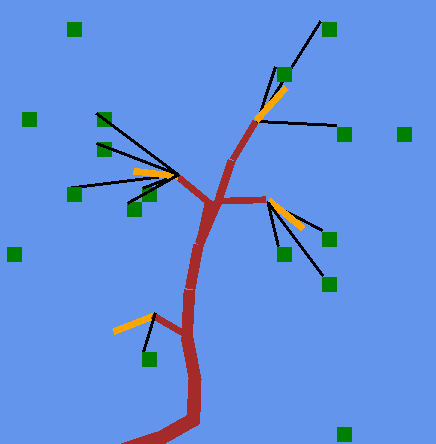The green squares are Leaves. The brown Branches are Branches that were grown in previous iterations. The black lines indicate each Leaf’s attraction to its nearest Branches (that are in range). The orange lines indicate the Branches that will grow in this iteration based on those attraction points.

The orange Branches will grow with respect to the original parent Branch GrowDirection, adding the attraction points average direction to this value.

Onto the code. See the bottom of the page for a download of a sample project for Visual Studio.

Leaf class:
```public class Leaf
{
public Vector2 Position { get; set; }
public Branch ClosestBranch { get; set; }

public Leaf(Vector2 position)
{
Position = position;
}
}
```
Branch class:
```public class Branch
{
public Branch Parent { get; private set; }
public Vector2 GrowDirection { get; set; }
public Vector2 OriginalGrowDirection { get; set; }
public int GrowCount { get; set; }
public Vector2 Position { get; private set; }

public Branch(Branch parent, Vector2 position, Vector2 growDirection)
{
Parent = parent;
Position = position;
GrowDirection = growDirection;
OriginalGrowDirection = growDirection;
}

public void Reset()
{
GrowCount = 0;
GrowDirection = OriginalGrowDirection;
}
}
```
Tree class:
```public class Tree
{
bool DoneGrowing = false;

Vector2 Position = Vector2.Zero;

int LeafCount = 400;
int TreeWidth = 80;
int TreeHeight = 150;
int TrunkHeight = 40;
int MinDistance = 2;
int MaxDistance = 15;
int BranchLength = 2;

Branch Root;
List<Leaf> Leaves;
Dictionary<Vector2, Branch> Branches;

Rectangle Crown;

public Tree(Vector2 position)
{
Position = position;
GenerateCrown();
GenerateTrunk();
}

private void GenerateCrown()
{
Crown = new Rectangle((int)Position.X - TreeWidth / 2, (int)Position.Y - TreeHeight - TrunkHeight, TreeWidth, TreeHeight);
Leaves = new List<Leaf>();

//randomly place leaves within our rectangle
for (int i = 0; i < LeafCount; i++)
{
Vector2 location = new Vector2(Random.Next(Crown.Left, Crown.Right + 1), Random.Next(Crown.Top, Crown.Bottom + 1));
Leaf leaf = new Leaf(location);
}
}

private void GenerateTrunk()
{
Branches = new Dictionary<Vector2, Branch>();

Root = new Branch(null, Position, new Vector2(0, -1));

Branch current = new Branch(Root, new Vector2(Position.X, Position.Y - BranchLength), new Vector2(0, -1));

//Keep growing trunk upwards until we reach a leaf
while ((Root.Position - current.Position).Length() < TrunkHeight)
{
Branch trunk = new Branch(current, new Vector2(current.Position.X, current.Position.Y - BranchLength), new Vector2(0, -1));
current = trunk;
}
}

public void Grow()
{
if (DoneGrowing) return;

//If no leaves left, we are done
if (Leaves.Count == 0) {
DoneGrowing = true;
return;
}

//process the leaves
for (int i = 0; i < Leaves.Count; i++)
{
bool leafRemoved = false;

Leaves[i].ClosestBranch = null;
Vector2 direction = Vector2.Zero;

//Find the nearest branch for this leaf
foreach (Branch b in Branches.Values)
{
direction = Leaves[i].Position - b.Position;                       //direction to branch from leaf
float distance = (float)Math.Round(direction.Length());            //distance to branch from leaf
direction.Normalize();

if (distance <= MinDistance)            //Min leaf distance reached, we remove it
{
Leaves.Remove(Leaves[i]);
i--;
leafRemoved = true;
break;
}
else if (distance <= MaxDistance)       //branch in range, determine if it is the nearest
{
if (Leaves[i].ClosestBranch == null)
Leaves[i].ClosestBranch = b;
else if ((Leaves[i].Position - Leaves[i].ClosestBranch.Position).Length() > distance)
Leaves[i].ClosestBranch = b;
}
}

//if the leaf was removed, skip
if (!leafRemoved)
{
//Set the grow parameters on all the closest branches that are in range
if (Leaves[i].ClosestBranch != null)
{
Vector2 dir = Leaves[i].Position - Leaves[i].ClosestBranch.Position;
dir.Normalize();
Leaves[i].ClosestBranch.GrowDirection += dir;       //add to grow direction of branch
Leaves[i].ClosestBranch.GrowCount++;
}
}
}

//Generate the new branches
HashSet<Branch> newBranches = new HashSet<Branch>();
foreach (Branch b in Branches.Values)
{
if (b.GrowCount > 0)    //if at least one leaf is affecting the branch
{
Vector2 avgDirection = b.GrowDirection / b.GrowCount;
avgDirection.Normalize();

Branch newBranch = new Branch(b, b.Position + avgDirection * BranchLength, avgDirection);

b.Reset();
}
}

//Add the new branches to the tree
foreach (Branch b in newBranches)
{
//Check if branch already exists.  These cases seem to happen when leaf is in specific areas
Branch existing;
if (!Branches.TryGetValue(b.Position, out existing))
{
}
}

//if no branches were added - we are done
//this handles issues where leaves equal out each other, making branches grow without ever reaching the leaf
DoneGrowing = true;
}
}
```
With further improvements and refinements, you can make the tree form any shape you want: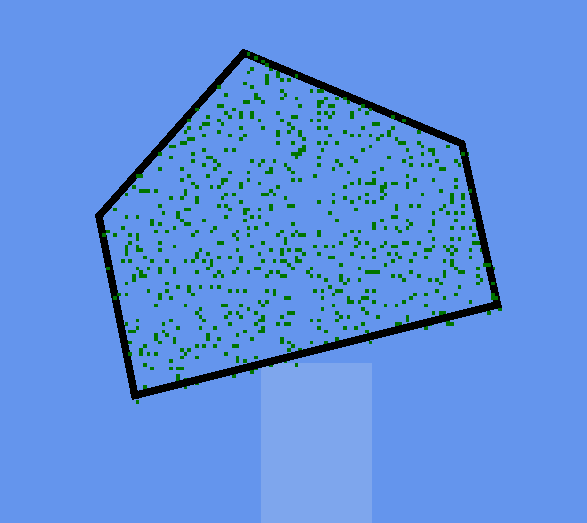Rendering some leaves: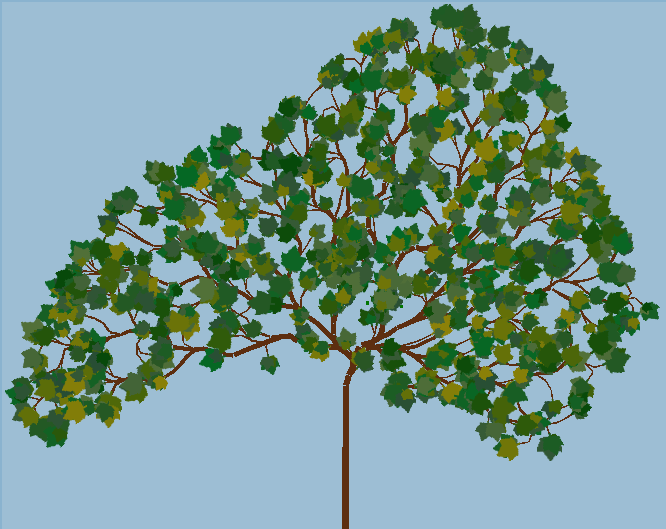Here is a gif to demonstrate what is possible with this method:
Tree Growing Gif

Cycling through the 4 seasons:
Season Cycle Gif

The demo project can be downloaded here. I have omitted the polygon code for the sake of simplicity, the trees are set to be Rectangles only.

http://www.jgallant.com/files/TreeGenerator.zip

Feel free to contact me if you have any questions or comments.

References used:
http://algorithmicbotany.org/papers/colonization.egwnp2007.html
http://procworld.blogspot.ca/2011/02/space-colonization.html
http://www.sea-of-memes.com/LetsCode26/LetsCode26.html